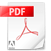Microwave Power Meter MPM26

Features:
* 10 MHz  to 26.5GHz  measurement range with USB.
* Digital Display on 320X240 Pixels Touchscreen TFT  with graph.
* Wide range from  +20dBm(100mW) to -30dBm(1uW)
* 0.1dB resolution
* Measurement in dBm & 22 other units
* Measure Gunn and Klystron Source outputs without PIN modulator. It can effectively replace the SWR meter for most experiments and allow measurements with unprecedented accuracy.

List of Experiments
1.To learn different ways of measuring power.
2.To evaluate the accuracy of the power measurements.
3.To plot the power output of Gunn/Klystron Oscillator with V
4.To plot the power output of a Gunn/Klystron Oscillator with frequency.
5. Study of square law modulation and square law characteristics of a crystal detector.
6. To measure PIN modulator insertion loss & mod. depth.
7. To measure the accuracy of SWR meter reading.
8. To calculate the relationship between Q and bandwidth of resonance cavity.
9.To measure the insertion loss of the waveguide.
10.To measure the insertion loss in the main line of a directional coupler.
11.To measure the coupling factor of a directional coupler.
12.To measure the isolation & directivity of a directional coupler.
13.To measure the return loss of a unknown load.
14.To measure the decoupling between H and E arms of magic Tee.
15.To measure the insertion loss of the hybrid Tee.
16. To measure the return loss of H arm in a magic Tee.
17.To measure and plot the attenuation characteristics of variable attenuator.
18.To measure the attenuation of a fixed attenuator.
19.To measure the input SWR of attenuator.
20.To measure the gain of a pyramidal horn.
21.To plot the E and H Plane polar pattern of a antenna and compute the beamwidth.
22.To measure the coupling coefficient of a waveguide E & H Plane Tee.
23.To measure the isolation of a E & H plane Tee.
24.To measure the input VSWR of a E & H plane Tee.
25.To study the operation of ferrite circulator and measure its insertion loss.
26.To measure isolation of a ferrite circulator.
27.To measure the cross coupling of a circulator.
28.To study the variation of characteristics of ferrite circulator with frequency.

## Video DemoMicrowave Power Meter MPM26 Datasheet
MPM26 26.5GHz Microwave Power Meter.pdf